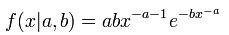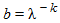AnyLogic

# gumbel2

In probability theory, the Type-2 Gumbel probability density function is:This implies that it is similar to the Weibull distributions, substitutingand a = − k. Note however that a positive k (as in the Weibull distribution) would yield a negative a, which is not allowed here as it would yield a negative probability density.

### gumbel2(double a, double b)

Description
Generates a sample of the Type-2 Gumbel distribution.
Parameters
Name Type of value Description
a double
b double
Result
Type Description
double The generated sample.

### gumbel2(double a, double b, java.util.Random r)

Description
Generates a sample of the Type-2 Gumbel distribution using the specified random number generator.
Parameters
Name Type of value Description
a double
b double
r java.util.Random The random number generator.
Result
Type Description
double The generated sample.